Previous: PISO Up: Algorithms PISO and SIMPLE Next: simpleFOAM Solver General Properties

This is an automatically generated documentation by LaTeX2HTML utility. In case of any issue, please, contact us at info@cfdsupport.com.

## SIMPLE

• Assume Navier-Stokes equations for steady-state incompressible fluid flow
• Mass conservation equation (continuity equation)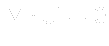(11.8)

• Momentum conservation equation (velocity equation)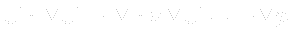(11.9)

• When we discretize momentum conservation equation, we get: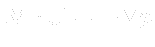(11.10)

• Matrix linear system: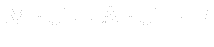(11.11)

• Matrix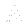is diagonal, with diagonal of original matrix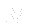•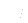is a vector corresponding to ()
• Rewriting () with substitution from ():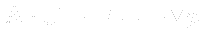(11.12)

• Express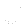from equation (), i.e. momentum correction equation :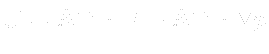(11.13)

• To momentum correction equation () applying continuity equation () getting: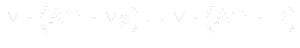(11.14)

• SIMPLE algorithm description:
1. Computation starts, initialand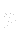are chosen
2. Time step starts
3. Boundary conditions are updated
4. Linear system is solved5. Relaxation6. Obtained new7. Building matrixand vector8. Mass flow over cell faces is computed
9. Pressure correction is applied ()
10. Relaxation11. Obtained new12. Mass flow over cell faces is corrected
13. Momentum correction is applied ()
14. Obtained new corrected15. Boundary conditions are updated
16. Steps 5 – 15 are repeated with respect to nCorrectors value
17. Time step ends

• Source code of SIMPLE algorithm is implemented e.g. for solver simpleFoam:
\$FOAM_SOLVERS/incompressible/simpleFoam